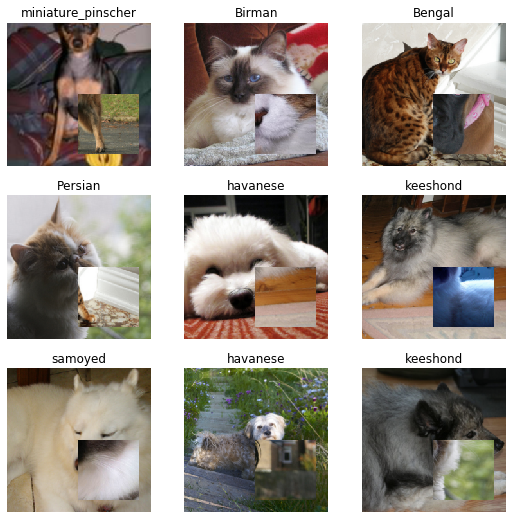Callback to apply CutMix data augmentation technique to the training data.

From the research paper, CutMix is a way to combine two images. It comes from MixUp and Cutout. In this data augmentation technique:

patches are cut and pasted among training images where the ground truth labels are also mixed proportionally to the area of the patches

Also, from the paper:> By making efficient use of training pixels and retaining the regularization effect of regional dropout, CutMix consistently outperforms the state-of-the-art augmentation strategies on CIFAR and ImageNet classification tasks, as well as on the ImageNet weakly-supervised localization task. Moreover, unlike previous augmentation methods, our CutMix-trained ImageNet classifier, when used as a pretrained model, results in consistent performance gains in Pascal detection and MS-COCO image captioning benchmarks. We also show that CutMix improves the model robustness against input corruptions and its out-of-distribution detection performances.

## classCutMix[source]

CutMix(alpha=1.0) :: Callback

Implementation of https://arxiv.org/abs/1905.04899

## How does the batch with CutMix data augmentation technique look like?

First, let's quickly create the dls using ImageDataLoaders.from_name_re DataBlocks API.

path = untar_data(URLs.PETS)
pat        = r'([^/]+)_\d+.*\$'
fnames     = get_image_files(path/'images')
item_tfms  = [Resize(256, method='crop')]
batch_tfms = [*aug_transforms(size=224), Normalize.from_stats(*imagenet_stats)]
dls = ImageDataLoaders.from_name_re(path, fnames, pat, bs=64, item_tfms=item_tfms,
batch_tfms=batch_tfms)


Next, let's initialize the callback CutMix, create a learner, do one batch and display the images with the labels. CutMix inside updates the loss function based on the ratio of the cutout bbox to the complete image.

cutmix = CutMix(alpha=1.)

learn  = cnn_learner(dls, resnet50, loss_func=CrossEntropyLossFlat(), cbs=cutmix, metrics=[accuracy, error_rate])
learn._do_begin_fit(1)
learn.epoch,learn.training = 0,True
learn.dl = dls.train
b = dls.one_batch()
learn._split(b)
learn('begin_batch')
_,axs = plt.subplots(3,3, figsize=(9,9))
dls.show_batch(b=(cutmix.x,cutmix.y), ctxs=axs.flatten())## Using CutMix in Training

learn = cnn_learner(dls, resnet50, loss_func=CrossEntropyLossFlat(), cbs=cutmix, metrics=[accuracy, error_rate])
# learn.fit_one_cycle(1)